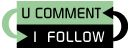## Friday, December 19, 2008

### Negative zero - what is it?

Computers have two ways of representing numbers:

• One is called sign and magnitude - usually you have one bit specifying the sign (again, most of time you have 0 for positive and 1 for negative) and the rest of the bits specify the absolute value ("magnitude") of the number.
• The other is ordering the numbers from the lowest to the highest (or the other way around) and specifying an index in this ordering - two's complement is for an example for this system, although it also has some nifty properties with regards to the arithmetic operations.

In the first case we can have a "+0" and a "-0" value. Now I'm no mathematician, so I checked the sources of knowledge :-). From the Mathworld article on Zero:

It is the only integer (and, in fact, the only real number) that is neither negative nor positive.

Furthermore, we have the following definition for the sign function:

The sign of a real number, also called sgn or signum, is -1 for a negative number (i.e., one with a minus sign "-"), 0 for the number zero, or +1 for a positive number (i.e., one with a plus sign "+"). In other words, for real x,

These lead me to believe that -0 and +0 are just an artifact of how we represent numbers in computers, and in fact they are one and the same entity. An additional proof is that IEEE 754 (the standard defining floating point representations - the most widely used sign and magnitude method to represent numbers) says in the standard:

5.11 Details of comparison predicates
...
Comparisons shall ignore the sign of zero (so +0 = −0)

So far, so good, right? Java has a small catch however:

Even though `-0.0 == 0.0`, `Double.valueOf(-0.0).compareTo(Double.valueOf(0.0))` is not zero (ie, the two objects are not equal)! This has wideraging implicatitions, one of the biggest being that if you use hashmaps or similar structrures with a Double key (given that you can't use double, because it isn't an object), they will show up as distinct entries! This may or may not be with what you want! One must mention that this behavior is clearly documented in the Java docs:

0.0d is considered by this method to be greater than -0.0d.

Then again, one must wonder how many people have read this document before running into the problem :-)

Contrasting with a few other programming languages:

• From the few tests I've done, it seems that .NET implements Double more intuitively (ie. `0 == Double.Parse("0.0").CompareTo(Double.Parse("-0.0"))`). This behavior is also consistent in collections (ie. they map to the same key in dictionaries), even though, when printed out, the two objects display the original signs. There also seems to be a (somewhat) complicated way to determine whether the given 0 is or is not zero.
• PHP (even though it doesn't have the same boxing / unboxing features) is consistent with the way .NET handles the situation: it prints -0 / 0 respectively, but they compare as equal and are considered the same key in associative arrays.
• In Perl, we have a behavior closer to Java: they compare as equal (again, no autoboxing), but in hashes they act as different keys.
• Python is again closer to .NET (they compare as equal and are considered the same key in associate arrays.
• Javascript also behaves the way .NET does (although there might be differences between the JS engine implementations of different browsers - I only tested it in FF3).
• Ruby and Smalltalk are left as exercises to the reader :-) (they should be interesting, since they both treat numbers as first class objects, meaning - that in a way - they are closer to Java or .NET than the other languages mentioned)

There are justifications for both approaches. On the one site, it is intuitive that -0 == +0, and breaking this expectation can introduce subtle errors in the programs. On the other side, the two objects are different (for example if you print them out, one will display -0.0 and the other 0.0) so (from this point of view) it is justified that they are not equal. Just make sure that you take this into account.